# WBJEE Maths Test - 11

## 75 Questions MCQ Test WBJEE Sample Papers, Section Wise & Full Mock Tests | WBJEE Maths Test - 11

Description
Attempt WBJEE Maths Test - 11 | 75 questions in 120 minutes | Mock test for JEE preparation | Free important questions MCQ to study WBJEE Sample Papers, Section Wise & Full Mock Tests for JEE Exam | Download free PDF with solutions
QUESTION: 1

Solution:
QUESTION: 2

### If (2r + 3)th and (r - 1)th terms in the expansion of (1 + x)15 have equal coefficients then r =

Solution: T2r + 3 = T2r + 2 + 1 = 15C2r + 2
Tr - 1 = Tr - 2 + 1 = 15Cr - 2
15C2r + 2 = 15Cr - 2
⇒ 2r + 2 + r - 2 = 15
⇒ r = 5
QUESTION: 3

### x2 + y2 + 2(2K+3)x - 2Ky +(2K+3)2 + K2 - r2 = 0 represents the family of circles with centres on the line

Solution:
QUESTION: 4

If the equation [(k(x+1)2/3)]+[(y+2)2/4]=1 represents a circle, then k=

Solution:
QUESTION: 5
For any complex number z, the minimum value of |z| + |z - 1| is
Solution:
QUESTION: 6

Let (1 + x)n =
C0 + C1x + C2x2 + ... + Cnxn and (C1/C0) + (2 C2/C1) + (3 C3/C2) + .... + ((n Cn)/(Cn - 1)) = (1/k)n(n + 1), then the value of k is

Solution: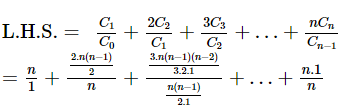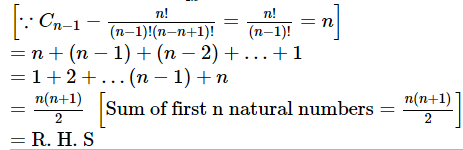QUESTION: 7
The maximum distance from the origin to the point z satisfying z+(1/z) = 2, is
Solution:
QUESTION: 8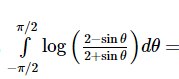Solution:
QUESTION: 9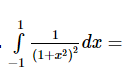Solution:
QUESTION: 10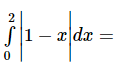Solution:
QUESTION: 11
If the system of equations x - ky - z = 0, kx - y - x = 0, x + y - z = 0 has a non-zero solution, then the possible values of k are
Solution:
QUESTION: 12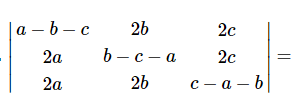Solution:
QUESTION: 13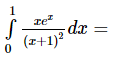Solution: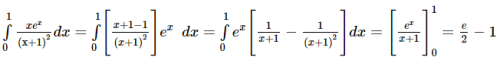QUESTION: 14

The solution of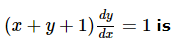Solution:

Put x + y + 1 = z
⇒ 1 + dy /dx = dz /dx ⇒ dy /dx = dz /dx - 1
(x + y + 1) dy dx = 1
⇒ z( dz /dx - 1) = 1 ⇒ dz /dx = 1 + 1 z ⇒ z z + 1 dz = dx ⇒ ∫(1 - 1 z + 1 )dz = ∫dx
⇒ z - log(z + 1) = x + c ⇒ x + y + 1 = log(x + y + 2) + x + c ⇒ y = log(x + y + 2) + log c
⇒ ey = (x + y + z)c ⇒ x + y + 2 = cey

QUESTION: 15

Solution of the differential equation tan y sec2 x dx + tan x sec2 y dy = 0 is

Solution:
QUESTION: 16
If x=a(t-sint) and y=a(1-cost), (dy/dx)=
Solution:
QUESTION: 17

If y=cot⁻1[(1+x)/(1-x)], (dy/dx)=

Solution:
QUESTION: 18

The latus rectum of the conic 3x2 + 4y2 - 6x + 8y - 5 = 0 is

Solution:
QUESTION: 19

If the length of the major axis of an ellipse is three times the length of its minor axis, then it's eccentricity is

Solution:
QUESTION: 20
The vertices of a hyperbola are (2, 0), (-2, 0) and the foci are (3, 0), (-3, 0). The equation of the hyperbola is
Solution:
QUESTION: 21

The eccentricity of the conic 9x2 - 16y2 = 144 is

Solution:
QUESTION: 22

In the interval (- 3,3) the function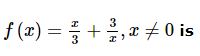Solution:
QUESTION: 23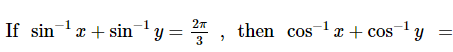Solution:
QUESTION: 24

The value of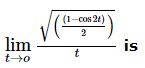Solution:
QUESTION: 25
If A, B are two square matrices such that AB = A and BA = B, then
Solution:
QUESTION: 26

The maximum area of the rectangle that can be inscribed in a circle of radius r is

Solution:
QUESTION: 27

If z2 = -i, then z =

Solution:
QUESTION: 28

The equation of the normal to the curve x2 = 4y at (1, 2) is

Solution:
QUESTION: 29

The length of the latus rectum of the parabola x2-4x-8y+12=0 is

Solution:
QUESTION: 30
In an election there are five candidates for three places. A man can vote for maximum number of candidates. In how many total ways can a men vote?
Solution:
QUESTION: 31

If nPr=840, nCr=35, then n=

Solution: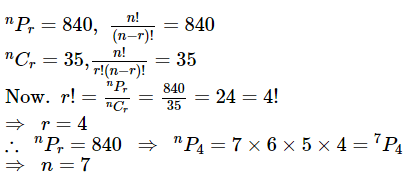QUESTION: 32
Three mangoes and three apples are in box. If two fruits are chosen at random, the probability that one is a mango and other is an apple is
Solution:
QUESTION: 33

Four numbers are chosen at random from {1, 2, 3, . . . , 40}. The probability that they are not consecutive is

Solution:
QUESTION: 34

A purse contains 4 copper coins and 3 silver coins, the second purse contains 6 copper coins and 2 silver coins. A coin is taken out from any purse. The probability that it is a copper coin is

Solution:
QUESTION: 35
If the ratio in angles of any ∆ABC 2:3:7 then the ratio is in its sides are
Solution:
QUESTION: 36
If a = 2, b = 3, c = 5 in triangle ABC, then C =
Solution:
QUESTION: 37

If two equations x2+a2=1-2ax and x2+b2=1-2bx have only one common root, then

Solution:
QUESTION: 38

If 2a + 3b + 6c = 0, then at least one root of the equation ax2+bx+c = 0 lies in the interval

Solution:
QUESTION: 39
If $f″\left(x\right)<0\forall x\in \left(a,b\right)$, then f'(x) = 0
Solution: Suppose, there are two points x1 and x2 in (a,b) such that f'(x1) = f'(x2) = 0. By Rolle's theorem applied to f' on [x1 , x2], there must then be a c $\in$ (x1, x2 ) such that f''(c) = 0. This contradicts the given condition $f″\left(x\right)<0\forall x\in \left(a,b\right)$ . Hence, our assumption is wrong. Therefore, there can be at most one point in (a,b) at which f'(x) is zero.
QUESTION: 40
If a, b, c are in A.P., then (a + 2b - c) (2b + c - a) ( c + a - b) equals
Solution:
QUESTION: 41
If (m + 1)th, (n + 1)th and (r + 1)th terms of an A.P. are in G.P. and m, n, r are in H.P., then ratio of the first term of the A.P. to its common diference in terms of n is
Solution:
QUESTION: 42

The fourth ,seventh and tenth of a G.P. are p,q,r respectively then

Solution:
QUESTION: 43
In a H.P.pth term is q and qth term p. Then the (pq)th term is
Solution:
QUESTION: 44
In Rule Method the null set is represented by
Solution:
QUESTION: 45
If A and B are matrices of order 2 such that A * B = (AB - BA)/2 then which of the following is false?
Solution:
QUESTION: 46
The opposite vertices of a square are (3,4) and (1,-1) then coordinates of rest vertices are
Solution:
QUESTION: 47

The equation of lines passing through the intersection of 4x-3y-1=0 and 2x-5y+3=0 which are equally inclined with axes, are

Solution:
QUESTION: 48

The number of roots of the equation |x|2-7|x|+12=0 is

Solution:
QUESTION: 49

If α , β are different values of x satisfying a cos x + b sin x = c then tan (α + β)  /2 =

Solution:
QUESTION: 50
The letters of the word COCHIN are permuted and all the permutations are arranged in an alphabetical order as in an English dictionary. The number of words that appear before the word COCHIN is
Solution:
QUESTION: 51

In how many different ways can the letters of the word DETAIL be arranged in such a way that the vowels occupy only the odd positions?

Solution:
QUESTION: 52

Let d 1 , d 2 , d 3 , … …, d k be all the divisors of a positive integer n including 1 and n.
Suppose d 1 + d 2 + d 3 + … … + d k = 72 , then the
value of 1 d 1 + 1 d 2 + 1 d 3 + … … + 1 d k is

Solution:
QUESTION: 53

For all x ∈ (0,1):

Solution:
QUESTION: 54

The value of tan− 1 e (i θ)is equal to

Solution:
QUESTION: 55

The value of cot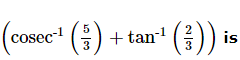Solution:
QUESTION: 56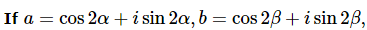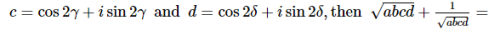Solution:
QUESTION: 57

For a positive integer n, let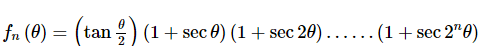Solution:
QUESTION: 58

Statement-1: For every natural number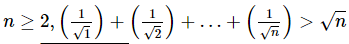Statement-2: For every natural number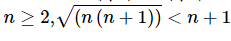Solution:
QUESTION: 59

Let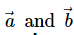be two unit vectors and α the angle between them. Vector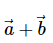→ will be a unit vector is α is equal to

Solution:
QUESTION: 60

The quadratic equations x2-6x+a=0 and x2-cx+6=0 have one root in common. The other roots of the first and second equations are integers in the ratio 4:3. Then the common root is

Solution:
QUESTION: 61

Let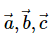be three non-zero vectors. Then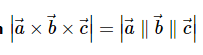if and only if

Solution:
QUESTION: 62

The value of p for which the function:
f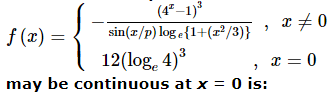Solution:
QUESTION: 63

Given P x = x 4 + a x 3 + b x 2 + c x + d such that x=0 is the only real root of P ′ x = 0. If P − 1 < P 1 , then in the interval [-1, 1]:

Solution:
QUESTION: 64

The number of seven digit integers, with sum of the digits equal to 10 and formed by using the digits 1,2 and 3 only, is

Solution:
QUESTION: 65

For which of the values of m the area of the region bounded by the curve y = x − x2 and y = mx equal 9 2 .

Solution:
*Multiple options can be correct
QUESTION: 66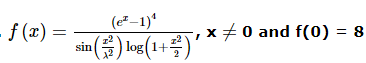be a continuous function, then λ is equal to

Solution:
*Multiple options can be correct
QUESTION: 67

Let a, b, c, d be real numbers such that
(a 2 + b 2 − 1 )(c 2 + d 2 − 1 )> (a c + b d − 1 )2
then which of the following statements is correct?

Solution:
*Multiple options can be correct
QUESTION: 68

If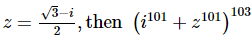equals

Solution:
*Multiple options can be correct
QUESTION: 69

For a positive interger n, let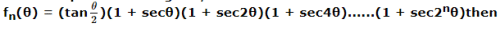Solution:
*Multiple options can be correct
QUESTION: 70

Let D be the domain of the function,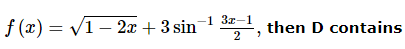Solution:
*Multiple options can be correct
QUESTION: 71

Let [x] = the greatest integer less than or equal to x. Then equation sinx = [1+sin x] +[1-cos x] has

Solution:
*Multiple options can be correct
QUESTION: 72

If 1, logyx, logzy, -15logxz are in A.P., then

Solution:
*Multiple options can be correct
QUESTION: 73

Let a 1, a 2, a 3, … … , a n n > 2 be real numbers such that a i = − a n − i + 1 for 1 ≤ i ≤ n and k = ∑ 1 ≤ i < j < k ≤ n ∑ ∑ x i x j x k , then which of the following is not true?

Solution:
*Multiple options can be correct
QUESTION: 74

The solutions of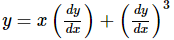are given by (where p = dy/dx and k is constant)

Solution:
*Multiple options can be correct
QUESTION: 75

If A = [ α β 0 α ] is the nth root of I2, then choose the correct statement

Solution:Use Code STAYHOME200 and get INR 200 additional OFF Use Coupon Code